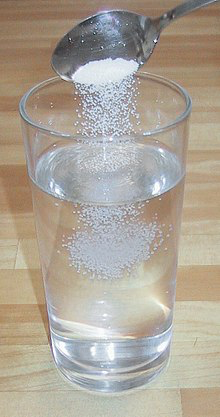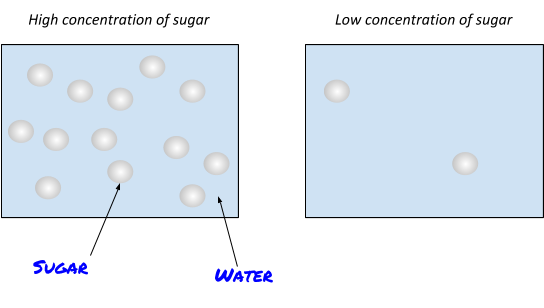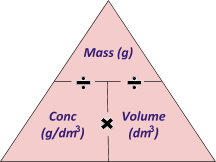# Concentrations

## Concentrations

Lots of substances that are used in chemistry are actually solutions. A solution is when a solute is dissolved in a solvent. For example, when sugar dissolves into water, the solute (sugar) is dissolved into the water (solvent) to form a solution (sugar water), yum!The amount of substance in a certain volume of a solution is called its concentration.

So a really sweet sugar solution would have a high concentration and a really weak sugar solution would have a low concentration.A very very very very useful concentration equation to remember (and understand) is:## Uncertainties in Measurements

All measurements have a level of uncertainty. For example, when you repeat an experiment 4 times, you may get 4 different (yet very similar) results.

Here is how to calculate the uncertainty of 5 experimental results (for this example, it doesn’t matter what the experiment was, you just need the numbers):First, you need to find the range of your results.

Find the largest value and subtract the smallest value from it: 2.5 - 2.2 = 0.3.

To find the uncertainty you just need to divide by 2!

0.3/2 = 1.5.

So the mean value would be 2.34 +- 1.5.